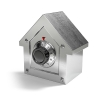#### You may also like### Always Two

Find all the triples of numbers a, b, c such that each one of them plus the product of the other two is always 2.### Symmetricality

Five equations and five unknowns. Is there an easy way to find the unknown values?### A Change in Code

Can you find the percentage increase if the results appear in code?

# Starting to Explore Four Consecutive Numbers

##### Age 11 to 16Challenge Level

Take four consecutive numbers, $a$, $b$, $c$, $d$.

1. (a) The four consecutive numbers sum to $130$. What are they?
(b) The four consecutive numbers sum to $-38$. What are they?

2. The sum of the first three consecutive numbers is $10$ more than the fourth. What are the four numbers?

3. What is $(a+d)-(b+c)$? Why?

4. Explore $a+b+c-d$.

If you enjoyed working on this problem, you may now want to take a look at the follow-up problem, Continuing to Explore Four Consecutive Numbers.

With thanks to Don Steward, whose ideas formed the basis of this problem.